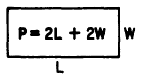Dependence, Functions, and FormulasCustom SearchCHAPTER 14 DEPENDENCE, FUNCTIONS, AND FORMULAS In chapter 13 of this course, use is made of several formulas, such as A = LW, E = IR, etc. It is the purpose of this chapter to explain the function and dependency relationships which make formulas so useful. DEPENDENCE AND FUNCTIONS Dependence may be defined as any relationship between two variables which allows the prediction of change in one of them as a result of change in the other. For example, the cost of 200 bolts depends upon the price per hundred. If C represents cost and p represents the price of 100 bolts, then the cost of 200 bolts may be expressed as follows: C = 2p In the example just given, C is called the DEPENDENT VARIABLE because its value depends upon the changing values of p. The INDEPENDENT VARIABLE is p. It is standard practice to isolate the dependent variable on the left side of an equation, as in the example. Consider the formula for the area of a rec- tangle, A = �LW. Here we. have two independent variables, L and W. Figure 14-1 (A) shows what happens if we double the length. Figure 14-1 (B) shows the result of doubling the width. Figure 14-1 (C!) shows the effect of doubling both length and width. Notice that when the length or width alone is doubled the area is doubled, but when both length and width are doubled the area is four times as great. In any equation showing a dependency relationship, the dependent variable is said to be a FUNCTION of the independent variable. Another use of the term "function" in describing an equation such as C = 2p is to refer to the whole expression as "the function C = 2p." This terminology is especially useful when the right-hand expression has several terms. For example, consider the equation y = 2x2 + 3x - 4. Mathematicians frequently use a shorthand notation and rewrite the equation as y = f(x). TheFigure 14-l.-Changes in the area of a rectangle resulting from changes in length and width. expression f(x) is understood to mean "a function of x" and reference to the function by calling it f(x) saves the space and time that would otherwise be required to write out all .three terms. Practice problems. Answer the following questions concerning the function r = d/t. 1. When t increases and d remains the same, does r: increase, decrease, or remain the same? 2. When d increases and t remains the same, does r increase, decrease, or remain the same ? 3. When t decreases and d remains the same, does r increase, decrease, or remain the same? 4. When d decreases and t remains the same, does r increase, decrease, or remain the same? 5. When d is doubled and t remains the same, is r doubled or halved? 6. When t is doubled and d remains the same, is r doubled or halved? Answers: 1. Decreases.  2. Increases.  3. Increases.  4. Decreases. 5. Doubled. 6. Halved. FORMULAS One of the most common uses of algebra is in the solution of formulas. Formulas have a wide and varied use throughout the Navy. It is important to know how formulas are derived, how to translate them into words, how to make them from word statements, and how to use them to solve problems. A formula is a general fact, rule, or principle expressed in algebraic symbols. It is a shorthand expression of a rule in which letters and signs of operation take the place of words. The formula always indicates the mathematical operations involved. For example, the formula P = 2L + 2W indicates that the perimeter (sum of the lengths of the sides) of a rectangle is equal to twice its length plus twice its width. (See fig. 14-2.)Figure 14-2.-Perimeter of a rectangle. A formula obtained by logical or mathematical reasoning is called a mathematical formula. A formula whose reliability is based on a limited number of observations, or on immediate experience, and not necessarily on established theories or laws is called an EMPIRICAL formula. Empirical formulas are found frequently in engineering and physical sciences. They sometimes are valid for only a limited number of values.Integrated Publishing, Inc. - A (SDVOSB) Service Disabled Veteran Owned Small Business